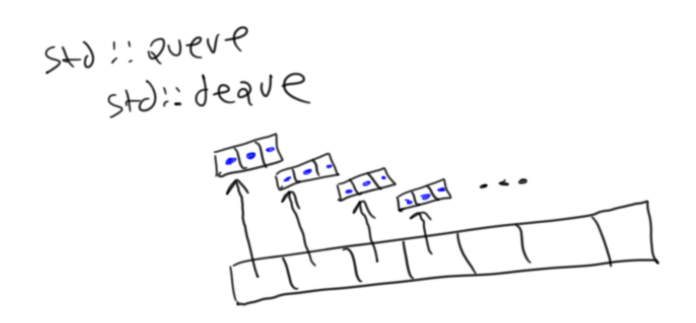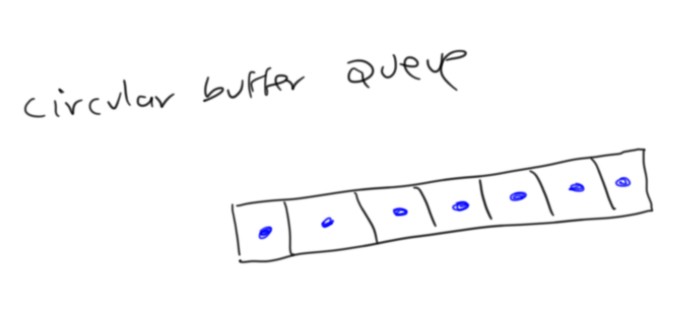• std::queue is a wrapper around std::deque, which is a double ended queue
• the implementation of std::deque has a dynamically allocated array of pointers, each of these pointers points to a fixed sized array. The items of the queue are stored in cells of these fixed size arrays
• this means that when you peek() or push() to std::queue/std::dequeue, you need to do 2 dereferences
• a circular buffer queue stores its items directly in the original array
• this means that peek() or push() operations on a circular buffer queue only has to do one dereference
• thus peek() and push() operations are faster on a circular buffer queue

I had a need for a queue recently in my physics engine, so I took a look into how std::queue (the standard queue in C++) was implemented in order to determine if it was fast enough for my use case.

std::queue is actually a wrapper around std::deque, which is a double ended queue. std::deque allows you to add and remove from both the front and the back of the queue, however std::queue only exposes adding to the back and removing from the front.

A std::deque has a dynamically allocated array of pointers, where each pointer points to a fixed size array (all fixed size arrays are of the same size). The items of the queue are actually stored in these fixed sized arrays.

Thus when you want to access (read or write) an item of std::deque, you need to do 2 dereferences. One you need to dereference to get the pointer, then you need to dereference to get the item in the fixed size array that the pointer points to.

Here is a graphical representation:A circular buffer queue is “flatter”. It stores the items directly in the original array, like so:This means that when a circular buffer has to access (read or write) to one of its cells, it only has to do one dereference.

The end result is that peek() and push() (i.e. read and write) operations should be faster in a circular buffer queue than std::deque.

But let’s prove this, via experimentation/measurement. I created a benchmark where I was push()ing a certain number of items to a queue. I then, one by one, peek()ed at the items and removed them. I did this for a varying number of items (one hundred, one thousand, 10 thousand, 100 thousand, all the way up to 100 million). I did this to both a std::deque and the circular buffer queue.

One thing to note is, I actually created two versions of the circular buffer queue. One that dynamically grows as items are added to it, and one that has a fixed size. The fixed size one has its size fixed upon initialization. The dynamically growable circular buffer has all the functionality of std::queue because it will grow as needed, however obviously, the fixed size circular buffer is more restricted, but I added it just for fun.

I wanted to know how much longer std::queue took than the circular buffer queue, so I plotted the ratio of time taken by std::queue over time taken by the circular buffer queue:This tells us that the circular buffer queue is about 1.3 times faster than std::queue.

Next, for fun, I wanted to know how much faster the fixed size version of the circular buffer was than std::queue, so I plotted the time ratio of std::queue to the fixed size circular buffer:As you can see, the fixed size circular buffer is about 1.5 times faster than std::queue.

Last, let’s see how much faster the fixed size circular buffer is than the non-fixed size buffer:It’s about 1.16 times faster (but of course it is more restricted because it has a fixed size).